Engineering ToolBox - Resources, Tools and Basic Information for Engineering and Design of Technical Applications!

# Solids - Volumes and Surfaces

## The volume and surface of solids like rectangular prism, cylinders, pyramids, cones and spheres - online calculator.

### Cube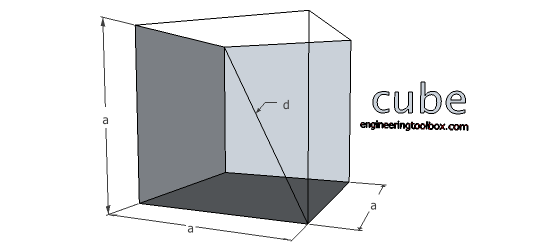#### Volume

V = a3                                (1)

where

V = volume (m3, ft3)

a = side (m, ft)

#### Surface Area

A0 = 6 a2                               (1b)

where

A0 = surface area (m2, ft2)

#### Diagonal

d = a 31/2                               (1c)

where

d = inside diagonal (m, ft)

#### Diagonal of Cube Face

ds = a 21/2                            (1d)

### Cuboid - Square Prism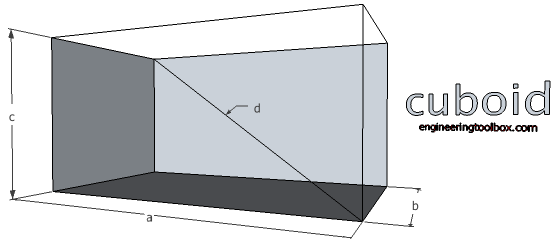#### Volume

V = a b c                                    (2)

where

V = volume of solid (m3, ft3)

a = length of rectangular prism (m, ft)

b = width of rectangular prism (m, ft)

c = height of rectangular prism (m, ft)

#### Diagonal

d =  (a2 + b2 + c2)1/2                                 (2b)

#### Surface Area

A0 = 2 (a b + a c + b c)                                   (2c)

where

A0 = surface area of solid (m2, ft2)

length

width

height

Volume:

Surface:

### Parallelepiped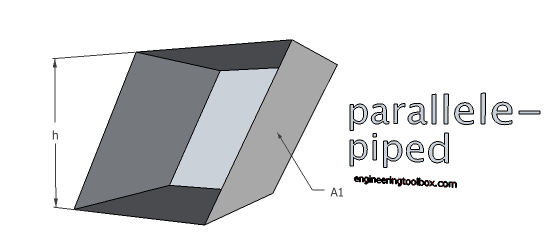#### Volume

V = A1 h                                    (3a)

where

A1 = side area (m2, ft2)

### Related Sketchup Components from The Engineering ToolBox•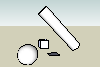Geometric Figures - Cylinders, Boxes, Cones, Planes, Spheres, Lines, Curves and more..

- free Engineering ToolBox plugin for use with the amazing Sketchup 3D drawing/model application.

### Cylinder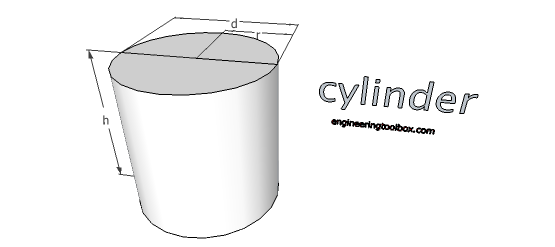#### Volume

V = π r2 h = (π / 4) d2                       (4a)

where

d = diameter of cylinder (m, ft)

r = radius of cylinder (m, ft)

h = height of cylinder (m, ft)

#### Surface

A = 2 π r h + 2 π r2                          (4b)

height

Volume:

Surface:

### Hollow Cylinder#### Volume

V = π/4 h (D2 - d2)                               (5)

### Pyramid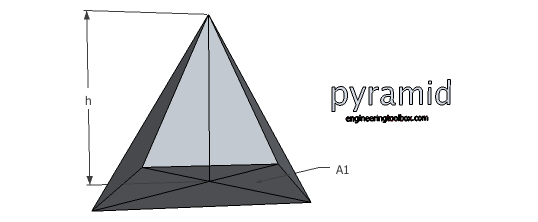#### Volume

V = 1/3 h A1                      (6)

where

A1 = area of base (m2, ft2)

h = perpendicular height of pyramid (m, ft)

#### Surface

A = ∑ sum of areas of triangles forming sides + Ab                           (6b)

where

the surface areas of the triangular faces will have different formulas for different shaped bases

area of base

perpendicular height

Volume:

### Frustum of Pyramid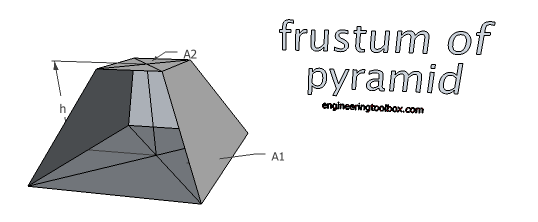### Volume

V = h/3 ( A1 + A2 + (A1 A2)1/2)                                     (7)

### Cone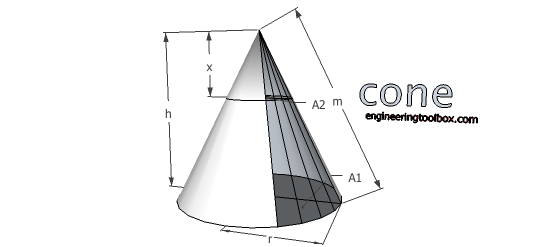#### Volume

V = 1/3 π r2 h                              (8a)

where

r = radius of cone base (m, ft)

h = height of cone (m, ft)

#### Surface

A = π r l + π r2                              (8b)

where

l = (r2 + h2)1/2 = length of cone side (m, ft)

height

Volume:

Surface:

#### Side

m = (h2 + r2)1/2                             (8c)

A2 / A1 = x2 / h2                             (8d)

### Frustum of Cone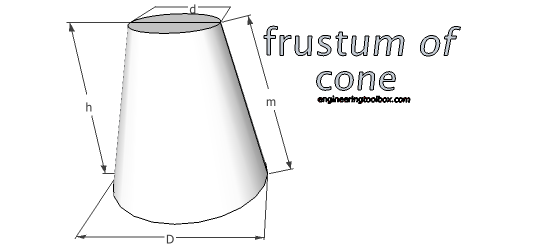#### Volume

V = π/12 h (D2 + D d + d2)                              (9a)

m = ( ( (D - d) / 2 )2 + h2)1/2                                (9c)

### Sphere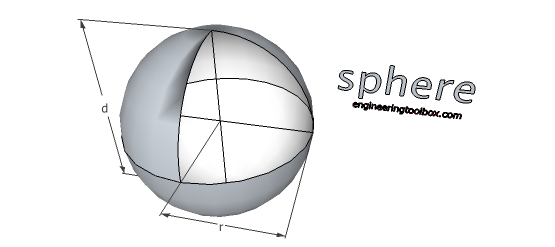#### Volume

V = 4/3 π r3

= 1/6 π d3                                    (10a)

where

r = radius of sphere (m, ft)

A = 4 π r2

= π d2     (10b)

Volume:

Surface:

#### Spheres with Fractional Diameters - Surface Area and Volume

Fraction Diameter
- d –
(inch)
Decimal Diameter
- d –
(inch)
– r –
(inch)
Surface Area
- A –
(in2)
Volume
- V -
(in3)
1/64 0.015625 0.007813 0.0007670 0.0000020
1/32 0.031250 0.015625 0.0030680 0.0000160
3/64 0.046875 0.023438 0.0069029 0.0000539
1/64 0.062500 0.031250 0.0122718 0.0001278
5/64 0.078125 0.039063 0.0191748 0.0002497
3/32 0.093750 0.046875 0.0276117 0.0004314
7/64 0.109375 0.054688 0.0375825 0.0006851
1/8 0.125000 0.062500 0.0490874 0.0010227
9/64 0.140625 0.070313 0.0621262 0.0014561
5/32 0.156250 0.078125 0.0766990 0.0019974
11/64 0.171875 0.085938 0.0928058 0.0026585
3/16 0.187500 0.093750 0.1104466 0.0034515
13/64 0.203125 0.101563 0.1296214 0.0043882
7/32 0.218750 0.109375 0.1503301 0.0054808
15/64 0.234375 0.117188 0.1725728 0.0067411
1/4 0.250000 0.125000 0.1963495 0.0081812
17/64 0.265625 0.132813 0.2216602 0.0098131
9/32 0.281250 0.140625 0.2485049 0.0116487
19/64 0.296875 0.148438 0.2768835 0.0137000
5/16 0.312500 0.156250 0.3067962 0.0159790
21/64 0.328125 0.164063 0.3382428 0.0184977
11/32 0.343750 0.171875 0.3712234 0.0212680
23/64 0.359375 0.179688 0.4057379 0.0243020
3/8 0.375000 0.187500 0.4417865 0.0276117
25/64 0.390625 0.195313 0.4793690 0.0312089
13/32 0.406250 0.203125 0.5184855 0.0351058
27/64 0.421875 0.210938 0.5591360 0.0393142
7/16 0.437500 0.218750 0.6013205 0.0438463
29/64 0.453125 0.226563 0.6450389 0.0487139
15/32 0.468750 0.234375 0.6902914 0.0539290
31/64 0.484375 0.242188 0.7370778 0.0595037
1/2 0.500000 0.250000 0.7853982 0.0654498
33/64 0.515625 0.257813 0.8352525 0.0717795
17/32 0.531250 0.265625 0.8866409 0.0785047
35/64 0.546875 0.273438 0.9395632 0.0856373
9/16 0.562500 0.281250 0.9940196 0.0931893
37/64 0.578125 0.289063 1.0500098 0.1011728
19/32 0.593750 0.296875 1.1075341 0.1095997
39/64 0.609375 0.304688 1.1665924 0.1184820
5/8 0.625000 0.312500 1.2271846 0.1278317
41/64 0.640625 0.320313 1.2893109 0.1376608
21/32 0.656250 0.328125 1.3529711 0.1479812
43/64 0.671875 0.335938 1.4181652 0.1588050
11/16 0.687500 0.343750 1.4848934 0.1701440
45/64 0.703125 0.351563 1.5531555 0.1820104
23/32 0.718750 0.359375 1.6229517 0.1944161
47/64 0.734375 0.367188 1.6942818 0.2073730
3/4 0.750000 0.375000 1.7671459 0.2208932
49/64 0.765625 0.382813 1.8415439 0.2349887
25/32 0.781250 0.390625 1.9174760 0.2496714
51/64 0.796875 0.398438 1.9949420 0.2649532
13/16 0.812500 0.406250 2.0739420 0.2808463
53/64 0.828125 0.414063 2.1544760 0.2973626
27/32 0.843750 0.421875 2.2365440 0.3145140
55/64 0.859375 0.429688 2.3201459 0.3323126
7/8 0.875000 0.437500 2.4052819 0.3507703
57/64 0.890625 0.445313 2.4919518 0.3698991
29/32 0.906250 0.453125 2.5801557 0.3897110
59/64 0.921875 0.460938 2.6698936 0.4102180
15/16 0.937500 0.468750 2.7611654 0.4314321
61/64 0.953125 0.476563 2.8539713 0.4533652
31/32 0.968750 0.484375 2.9483111 0.4760294
63/64 0.984375 0.492188 3.0441849 0.4994366
1 1.000000 0.500000 3.1415927 0.5235988

### Zone of a Sphere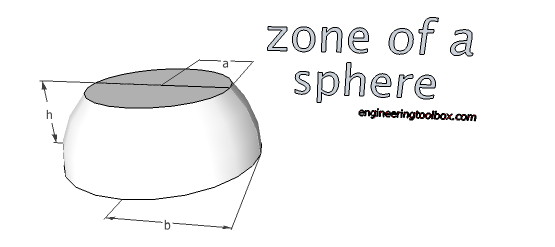V = π/6 h (3a2 + 3b2 + h)                             (11a)

Am = 2 π r h    (11b)

A0 = π (2 r h + a2 + b2)                               (11c)

### Segment of a Sphere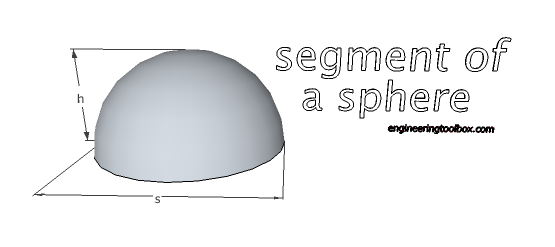V = π/6 h (3/4 s2 + h2

=   π h2 (r - h/3)                                  (12a)

Am = 2 π r h

π/4 (s2 + 4 h2)                               (12b)

### Sector of a Sphere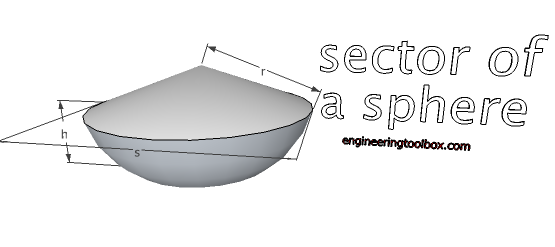V = 2/3 π r2 h                            (13a)

A0 = π/2 r (4 h + s)                          (13b)

### Sphere with Cylindrical Boring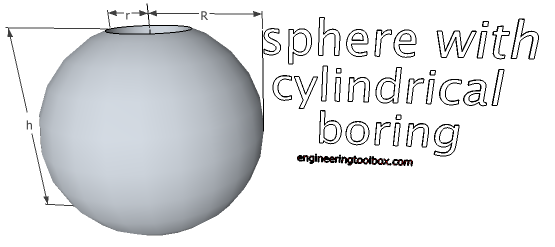V = π/6  h3                               (14a)

A0 = 4 π ((R + r)3 (R - r))1/2

= 2 π h (R + r)                                (14b)

h = 2 (R2 - r2)1/2                                 (14c)

### Sphere with Conical Boring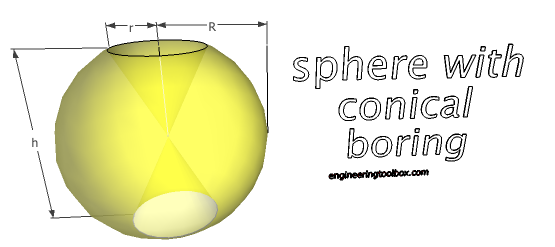V = 2/3 π R2 h                           (15a)

A0 = 2 π R (h + (R2 - h2/4)1/2)                              (15b)

h = 2 (R2 - r2)1/2                           (15c)

### Torus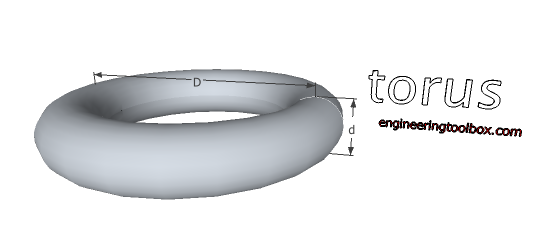V = π2/4 D d2                           (16a)

A0 = π2 D d                            (16b)

### Sliced Cylinder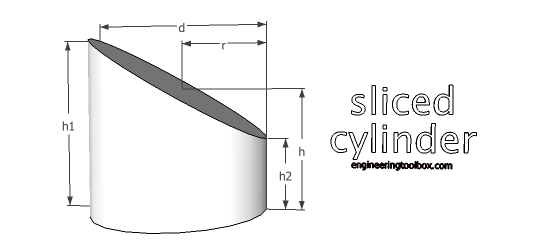V = π/4 d2 h

= π r2 ((h1 + h2) / 2)                           (17a)

Am = π d h

= 2 π r ((h1 + h2) / 2)                          (17b)

where

Am = side walls area

A0 = π r (h1 + h2 + r + (r2 + (h1 - h2)2/4)1/2)                                (17c)

where

A0 = surface area

### Ungula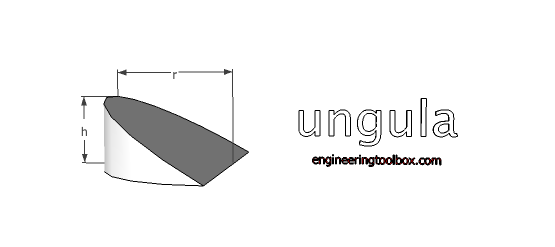V = 2/3 r2 h            (18a)

Am = 2 r h              (18b)

A0 = Am + π/2 r2 + π/2 r (r2 + h2)1/2                                      (18c)

### Barrel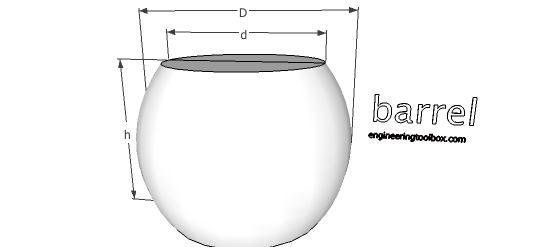V ≈ π/12 h (2 D2 + d2)                                  (19a)

## Related Topics

• ### Basics

The SI-system, unit converters, physical constants, drawing scales and more.
• ### Mathematics

Mathematical rules and laws - numbers, areas, volumes, exponents, trigonometric functions and more.

## Related Documents

• ### Area Units Converter

Convert between units of area.
• ### Centroids of Plane Areas

The controid of square, rectangle, circle, semi-circle and right-angled triangle.
• ### Cooking Units Volume Converter - US and Metric Units

Convert between cooking liquid and dry measuring US and metric volume units.
• ### Cooking Volume Units Converter

Convert between different liquid cooking volume units.
• ### Cylindrical Tanks - Volumes

Volume in US gallons and liters.
• ### Equal Areas - Circles vs. Squares

Radius and side lengths of equal areas, circles and squares.
• ### Mild Steel - Round Bars Weight

Weight of round bars.
• ### Pipes with Water Content - Weight and Volume

Estimate water content in pipes - weight and volume.
• ### Rectangular Tanks - Volumes

Tank volume per foot depth.
• ### Solids - Melting and Boiling Temperatures

Melting and boiling temperatures of some products.
• ### Squaring with Diagonal Measurements

A rectangle is square if the lengths of both diagonals are equal.
• ### Volume Units Converter

Convert between common volume units like cubic metre, cubic feet, cubic inches and more.

## Engineering ToolBox - SketchUp Extension - Online 3D modeling!

Add standard and customized parametric components - like flange beams, lumbers, piping, stairs and more - to your Sketchup model with the Engineering ToolBox - SketchUp Extension - enabled for use with older versions of the amazing SketchUp Make and the newer "up to date" SketchUp Pro . Add the Engineering ToolBox extension to your SketchUp Make/Pro from the Extension Warehouse !

We don't collect information from our users. More about

## Citation

• The Engineering ToolBox (2008). Solids - Volumes and Surfaces. [online] Available at: https://www.engineeringtoolbox.com/surface-volume-solids-d_322.html [Accessed Day Month Year].

Modify the access date according your visit.

9.19.12